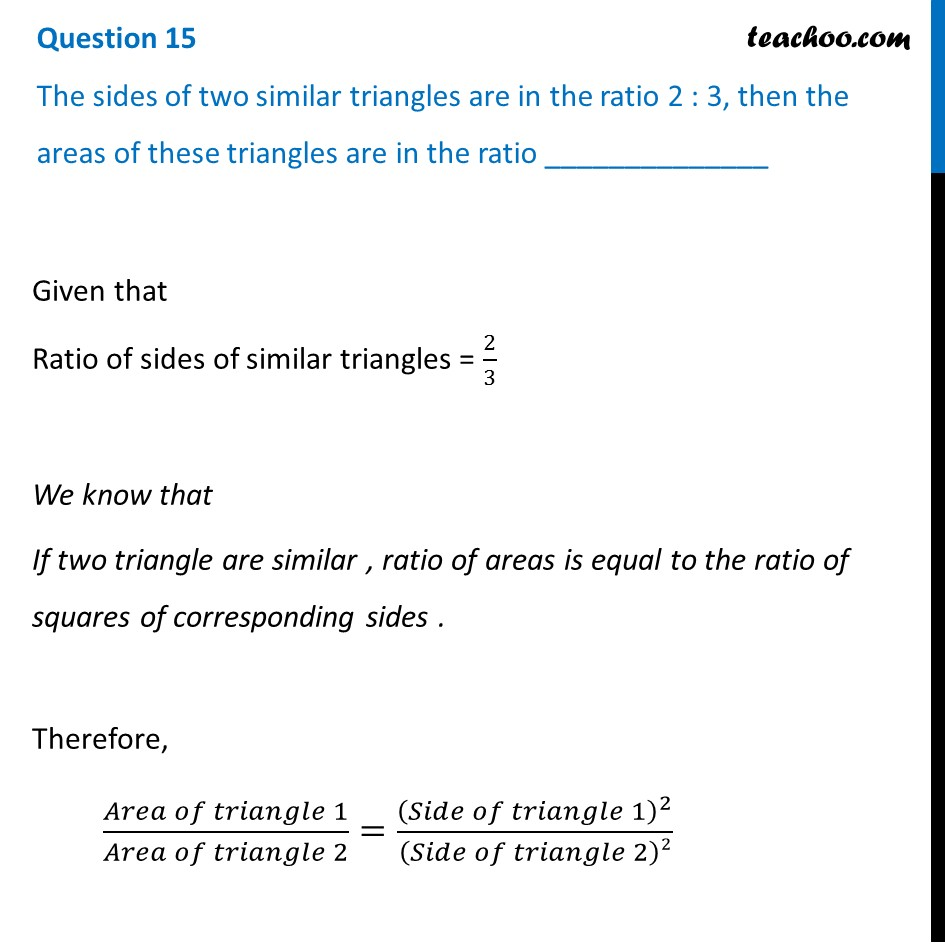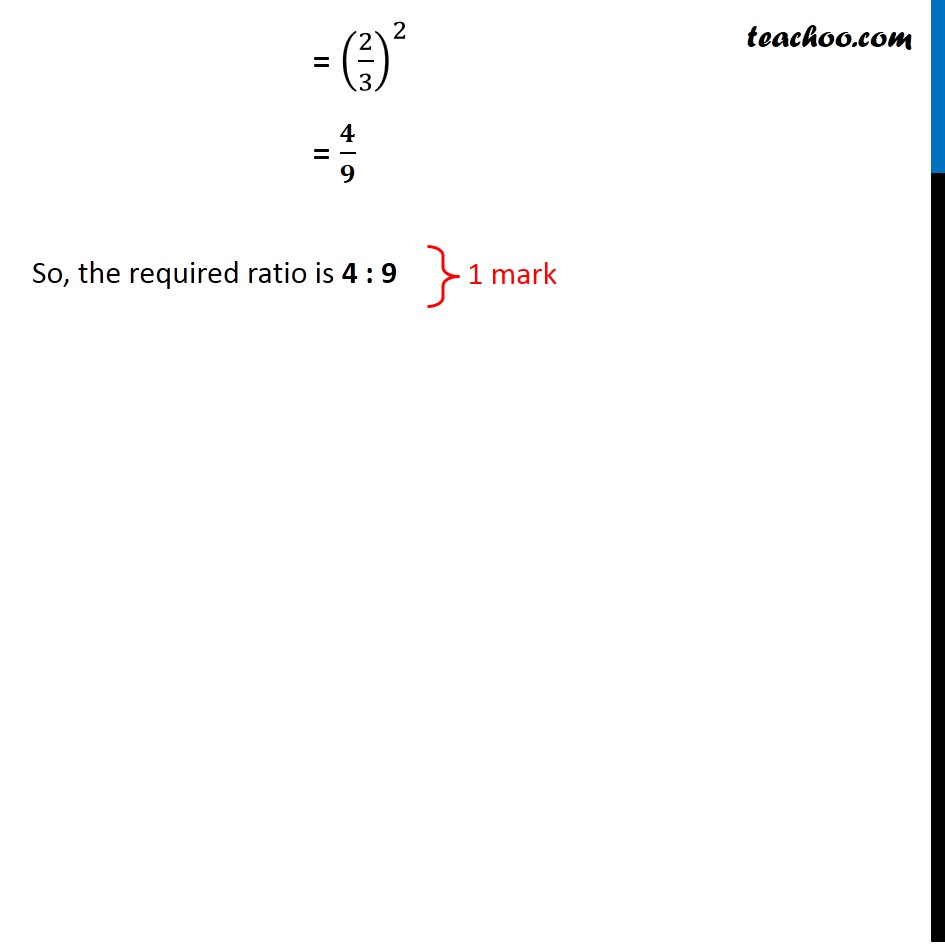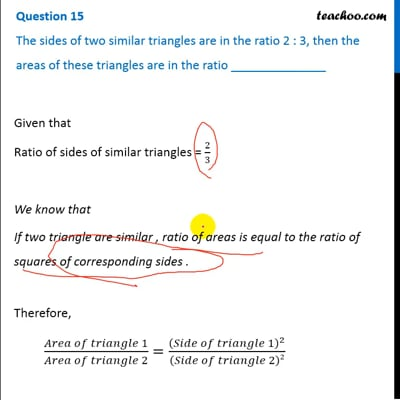CBSE Class 10 Sample Paper for 2020 Boards - Maths Basic

Class 10
Solutions of Sample Papers for Class 10 Boards

## The sides of two similar triangles are in the ratio 2:3, then the areas of these triangles are in the ratio ______________This video is only available for Teachoo black users

### Transcript

The sides of two similar triangles are in the ratio 2 : 3, then the areas of these triangles are in the ratio ______________ Given that Ratio of sides of similar triangles = 2/3 We know that If two triangle are similar , ratio of areas is equal to the ratio of squares of corresponding sides . Therefore, (𝐴𝑟𝑒𝑎 𝑜𝑓 𝑡𝑟𝑖𝑎𝑛𝑔𝑙𝑒 1)/(𝐴𝑟𝑒𝑎 𝑜𝑓 𝑡𝑟𝑖𝑎𝑛𝑔𝑙𝑒 2)=(𝑆𝑖𝑑𝑒 𝑜𝑓 𝑡𝑟𝑖𝑎𝑛𝑔𝑙𝑒 1)^2/(𝑆𝑖𝑑𝑒 𝑜𝑓 𝑡𝑟𝑖𝑎𝑛𝑔𝑙𝑒 2)2 = (2/3)^2 = 𝟒/𝟗 So, the required ratio is 4 : 9